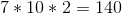# ISEE Middle Level Math : How to find the square root

## Example Questions

### Example Question #21 : Squares / Square Roots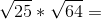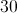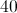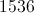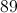Explanation:

First, find the square roots for each: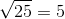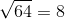Then, solve accordingly: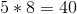### Example Question #21 : Squares / Square Roots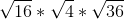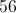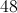Explanation:

First find the square roots: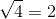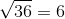Then, solve accordingly: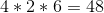### Example Question #23 : Squares / Square Roots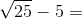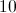Explanation:

First, find the square root:Then, solve accordingly: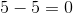### Example Question #24 : Squares / Square Roots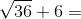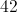Explanation:

First, find the square root:Then, solve accordingly: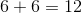### Example Question #25 : Squares / Square Roots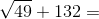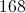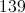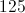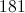Explanation:

First, find the sqauare root: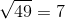Then, solve accordingly: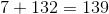### Example Question #26 : Squares / Square Roots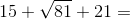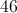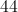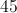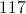Explanation:

First, find the square root: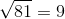Then, solve the equation: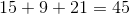### Example Question #27 : Squares / Square Roots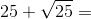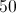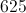Explanation:

First, find the square root:Then, solve: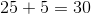### Example Question #28 : Squares / Square Roots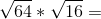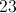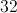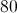Explanation:

First, find the square roots: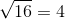Then, solve: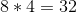### Example Question #29 : Squares / Square Roots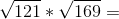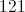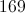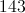Explanation:

First, find the square roots: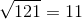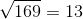Then, solve: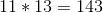### Example Question #21 : How To Find The Square Root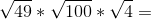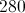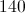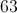Explanation:

First, find the square roots: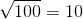Then, solve: